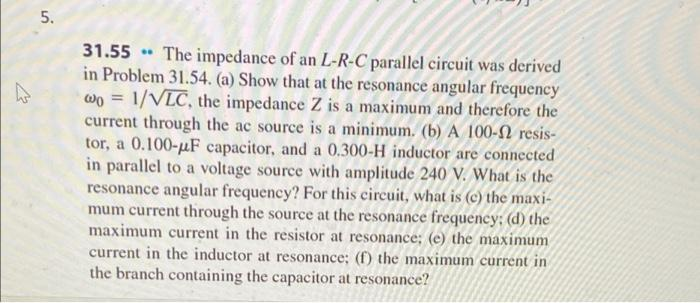# Question 5. N 31.55 The impedance of an L-R-C parallel circuit was derived in Problem 31.54. (a) Show that at the resonance angular frequency wo = 1/VLC, the impedance Z is a maximum and therefore the current through the ac source is a minimum. (b) A 100-12 resis- tor, a 0.100-uF capacitor, and a 0.300-H inductor are connected in parallel to a voltage source with amplitude 240 V. What is the resonance angular frequency? For this circuit, what is (c) the maxi- mum current through the source at the resonance frequency: (d) the maximum current in the resistor at resonance; (e) the maximum current in the inductor at resonance; (f) the maximum current in the branch containing the capacitor at resonance?XOWP3X The Asker · Mechanical EngineeringTranscribed Image Text: 5. N 31.55 The impedance of an L-R-C parallel circuit was derived in Problem 31.54. (a) Show that at the resonance angular frequency wo = 1/VLC, the impedance Z is a maximum and therefore the current through the ac source is a minimum. (b) A 100-12 resis- tor, a 0.100-uF capacitor, and a 0.300-H inductor are connected in parallel to a voltage source with amplitude 240 V. What is the resonance angular frequency? For this circuit, what is (c) the maxi- mum current through the source at the resonance frequency: (d) the maximum current in the resistor at resonance; (e) the maximum current in the inductor at resonance; (f) the maximum current in the branch containing the capacitor at resonance?
More
Transcribed Image Text: 5. N 31.55 The impedance of an L-R-C parallel circuit was derived in Problem 31.54. (a) Show that at the resonance angular frequency wo = 1/VLC, the impedance Z is a maximum and therefore the current through the ac source is a minimum. (b) A 100-12 resis- tor, a 0.100-uF capacitor, and a 0.300-H inductor are connected in parallel to a voltage source with amplitude 240 V. What is the resonance angular frequency? For this circuit, what is (c) the maxi- mum current through the source at the resonance frequency: (d) the maximum current in the resistor at resonance; (e) the maximum current in the inductor at resonance; (f) the maximum current in the branch containing the capacitor at resonance?